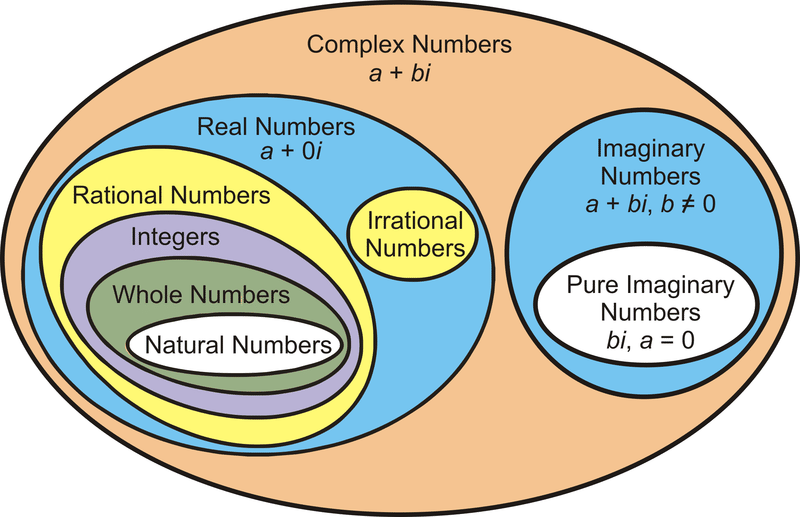6,241 views

What is the correct translation of the following statement into mathematical logic?

“Some real numbers are rational”

1. $\exists x (\text{real}(x) \lor \text{rational}(x))$
2. $\forall x (\text{real}(x) \to \text{rational}(x))$
3. $\exists x (\text{real}(x) \wedge \text{rational}(x))$
4. $\exists x (\text{rational}(x) \to \text{real}(x))$

just a Hint Use ^ for there exist(∃)
for ALL

∀x(rational(x)→real(x))

Meaning of each choices:

1. There exists a number which is either real or rational
2. If a number is real it is rational
3. There exists a number which is real and rational
4. There exists a number such that if it is rational, it is real

So, (C) is the answer.

by

what is the problem in option d,

it also conveys the same meaning i guess;

there exists a number such that if it is rational then real.

and question also says that some real numbers are rational numbers.

where am i wrong??

Hi @Ayush Upadhyaya sir,

I get confused when to translate a statement as AND (^) and when to use implication (-->) .

eg. None of my friends are  perfect... here should i consider Friends ^ Perfect  or Friends-->Perfect

For statement : Good mobile phones are not cheap.  (from gate 2014) i used implication and that leads to the right answer.

Similarly Not all that glitters is gold(2014) also implication gives the correct result

Any tips or any source to refer pls

suppose the number is complex so real(x) is false but real(x) → rational(x) will be true but rational numbers are not complex

“ There exists a number which is real and rational” and this is equal to “Some real number are rational number.”Rational numbers are always real

If possible, can you please draw the ven diagrams for other options given in question ? It might be helpful. @LakshmanPatelRJIT

You can try it out.Please, check and correct if wrong.

https://gateoverflow.in/?qa=blob&qa_blobid=4820487837657352897

And which tool you used for image ? Don’t know why my image is rotated. :)

(C) is the answer.

Translation of (C):" There exists a number which is real and rational " and this is eqt to “Some real numbers are rational”.
by